Equivalent Fractions Math Video for Kids | 3rd, 4th & 5th Grade
1%
It was processed successfully!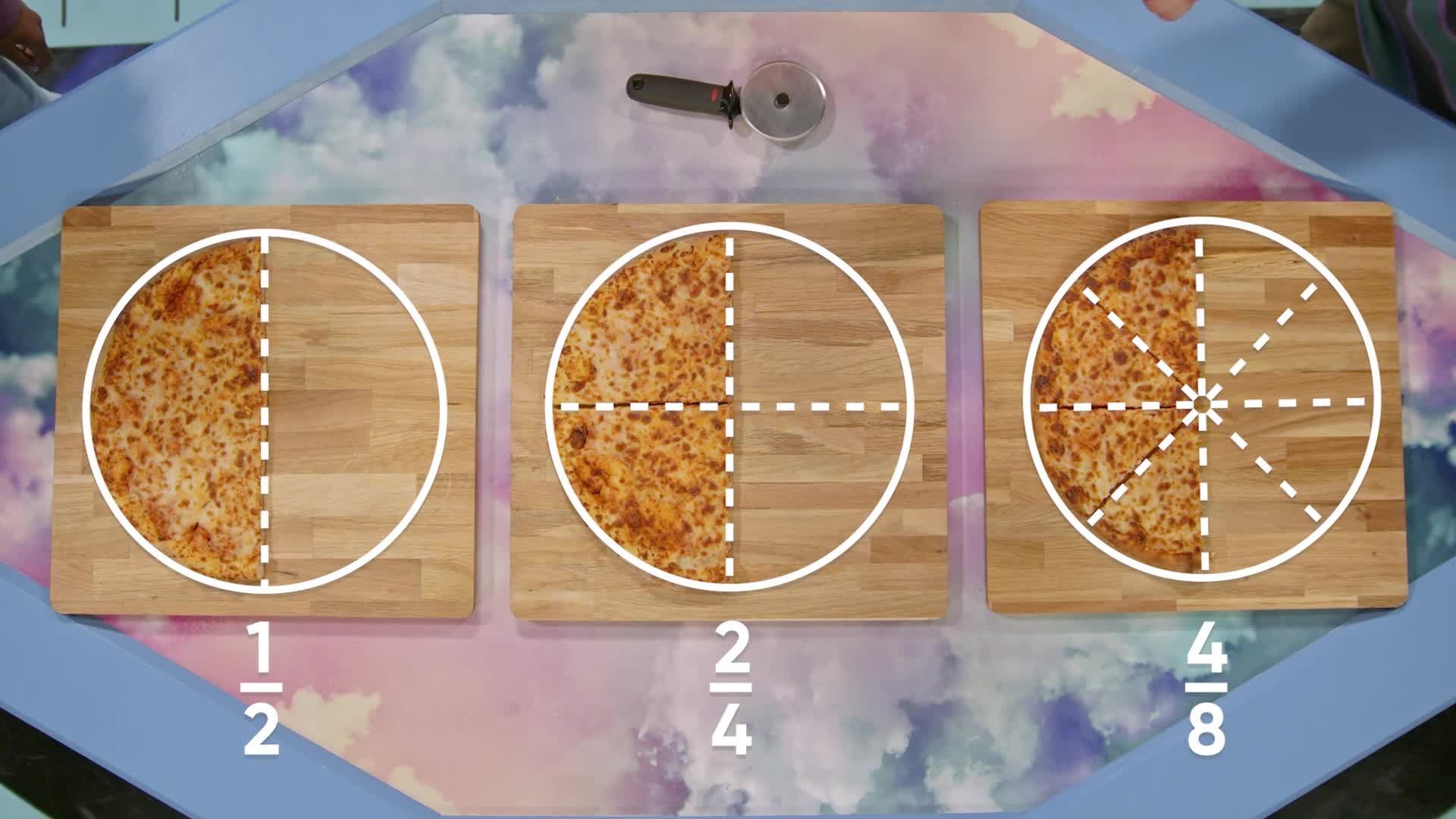WHAT ARE EQUIVALENT FRACTIONS?

Equivalent fractions are fractions that represent the same part of a whole. You can use multiplication and division to make equivalent fractions.

To better understand equivalent fractions…

WHAT ARE EQUIVALENT FRACTIONS?. Equivalent fractions are fractions that represent the same part of a whole. You can use multiplication and division to make equivalent fractions. To better understand equivalent fractions…

## LET’S BREAK IT DOWN!

### Pizza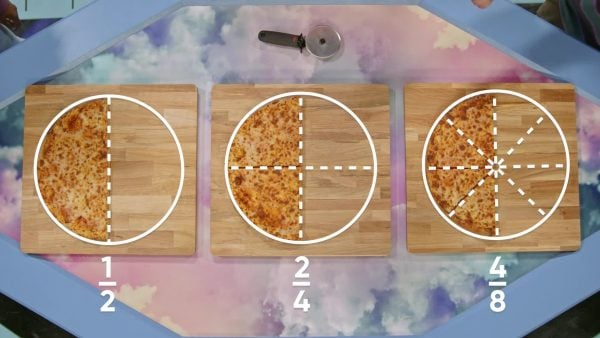April, Adesina, and Marcos have leftover pizza. Everyone's pizza started out the same size and shape. April’s pizza was cut into 2 equal pieces, and she has one piece left. This is represented with [ggfrac]1/2[/ggfrac]. Adesina’s pizza was cut into 4 equal pieces and she has 2 pieces left. This is represented with [ggfrac]2/4[/ggfrac]. Marcos’ pizza was cut into 8 equal pieces and he has 4 pieces left. This is represented with [ggfrac]4/8[/ggfrac]. Who has more pizza left? Visually, it looks like all three have the same amount of pizza, but what’s the difference? Even though the fractions have different numerators and denominators, they represent the same amount of a whole pizza. They are called equivalent fractions. We prove this using a pizza cutter: if we double the number of slices by cutting April’s slice in half, we turn [ggfrac]1/2[/ggfrac] into [ggfrac]2/4[/ggfrac]. If we again double the number of slices by cutting each [ggfrac]1/4[/ggfrac] in half, we turn [ggfrac]2/4[/ggfrac] into [ggfrac]4/8[/ggfrac]. Now you try: Draw a pizza that has [ggfrac]2/3[/ggfrac] left. Draw lines to show that this is the same amount as [ggfrac]4/6[/ggfrac].

Pizza April, Adesina, and Marcos have leftover pizza. Everyone's pizza started out the same size and shape. April’s pizza was cut into 2 equal pieces, and she has one piece left. This is represented with [ggfrac]1/2[/ggfrac]. Adesina’s pizza was cut into 4 equal pieces and she has 2 pieces left. This is represented with [ggfrac]2/4[/ggfrac]. Marcos’ pizza was cut into 8 equal pieces and he has 4 pieces left. This is represented with [ggfrac]4/8[/ggfrac]. Who has more pizza left? Visually, it looks like all three have the same amount of pizza, but what’s the difference? Even though the fractions have different numerators and denominators, they represent the same amount of a whole pizza. They are called equivalent fractions. We prove this using a pizza cutter: if we double the number of slices by cutting April’s slice in half, we turn [ggfrac]1/2[/ggfrac] into [ggfrac]2/4[/ggfrac]. If we again double the number of slices by cutting each [ggfrac]1/4[/ggfrac] in half, we turn [ggfrac]2/4[/ggfrac] into [ggfrac]4/8[/ggfrac]. Now you try: Draw a pizza that has [ggfrac]2/3[/ggfrac] left. Draw lines to show that this is the same amount as [ggfrac]4/6[/ggfrac].

### Dividing Clay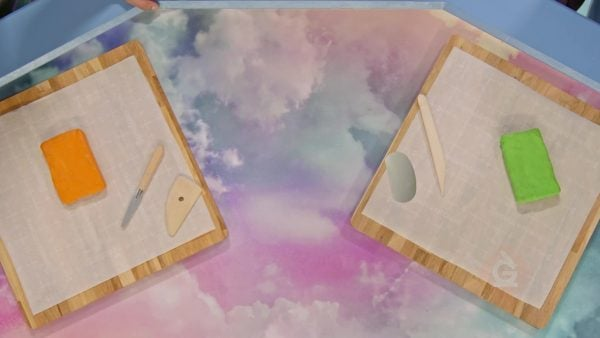April and Marcos have a block of clay to use for an art project. April wants to use [ggfrac]1/2[/ggfrac] of the block, while Marcos wants to use [ggfrac]8/16[/ggfrac] of the block. How can we find out if these amounts are the same? We start by cutting the block into two halves. Now we can show what [ggfrac]1/2[/ggfrac] looks like. Next, cut each of the pieces in half to show what [ggfrac]2/4[/ggfrac] looks like. If we repeat this step, we can show what [ggfrac]4/8[/ggfrac] looks like, and finally, what [ggfrac]8/16[/ggfrac] looks like. We can represent all of these pieces using the same amount of clay, so [ggfrac]1/2[/ggfrac], [ggfrac]2/4[/ggfrac], [ggfrac]4/8[/ggfrac], and [ggfrac]8/16[/ggfrac] are all equivalent fractions. To make equivalent fractions using math, we multiply both the denominator and the numerator by 2 each time. For [ggfrac]1/2[/ggfrac], we multiply 1 × 2 = 2, and 2 × 2 = 4 to get [ggfrac]2/4[/ggfrac]. They are equivalent. Each time we double the numerator, we must double the denominator as well to get an equivalent fraction. In fact, we can find an equivalent fraction by multiplying the numerator and denominator by any number, as long as it is the same number. Now you try: Find 3 fractions that are equivalent to [ggfrac]1/3[/ggfrac].

Dividing Clay April and Marcos have a block of clay to use for an art project. April wants to use [ggfrac]1/2[/ggfrac] of the block, while Marcos wants to use [ggfrac]8/16[/ggfrac] of the block. How can we find out if these amounts are the same? We start by cutting the block into two halves. Now we can show what [ggfrac]1/2[/ggfrac] looks like. Next, cut each of the pieces in half to show what [ggfrac]2/4[/ggfrac] looks like. If we repeat this step, we can show what [ggfrac]4/8[/ggfrac] looks like, and finally, what [ggfrac]8/16[/ggfrac] looks like. We can represent all of these pieces using the same amount of clay, so [ggfrac]1/2[/ggfrac], [ggfrac]2/4[/ggfrac], [ggfrac]4/8[/ggfrac], and [ggfrac]8/16[/ggfrac] are all equivalent fractions. To make equivalent fractions using math, we multiply both the denominator and the numerator by 2 each time. For [ggfrac]1/2[/ggfrac], we multiply 1 × 2 = 2, and 2 × 2 = 4 to get [ggfrac]2/4[/ggfrac]. They are equivalent. Each time we double the numerator, we must double the denominator as well to get an equivalent fraction. In fact, we can find an equivalent fraction by multiplying the numerator and denominator by any number, as long as it is the same number. Now you try: Find 3 fractions that are equivalent to [ggfrac]1/3[/ggfrac].

### Sandwich Deli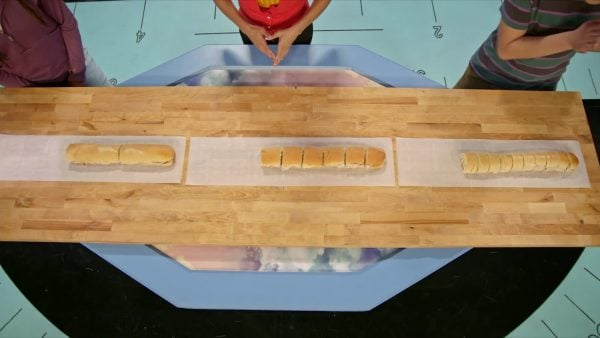Adesina shows April and Marcos how comparing equivalent fractions helps us serve lunch. How can we check if [ggfrac]2/3[/ggfrac] is equal to [ggfrac]6/9[/ggfrac] or [ggfrac]10/15[/ggfrac]? Start with [ggfrac]2/3[/ggfrac]. If we multiply the numerator and denominator by 2, we get [ggfrac]4/6[/ggfrac], so it is an equivalent fraction. If we instead multiply the numerator and denominator by 3, we get [ggfrac]6/9[/ggfrac]. [ggfrac]6/9[/ggfrac] is an equivalent fraction. Multiplying by 4, we get [ggfrac]8/12[/ggfrac], and multiplying by 5, we get [ggfrac]10/15[/ggfrac]. All of these fractions are equivalent, and we can find an infinite number of equivalent fractions, multiplying both the numerator and denominator by the same number. Now you try: Is [ggfrac]2/5[/ggfrac] equivalent to [ggfrac]14/35[/ggfrac]? How do you know?

Sandwich Deli Adesina shows April and Marcos how comparing equivalent fractions helps us serve lunch. How can we check if [ggfrac]2/3[/ggfrac] is equal to [ggfrac]6/9[/ggfrac] or [ggfrac]10/15[/ggfrac]? Start with [ggfrac]2/3[/ggfrac]. If we multiply the numerator and denominator by 2, we get [ggfrac]4/6[/ggfrac], so it is an equivalent fraction. If we instead multiply the numerator and denominator by 3, we get [ggfrac]6/9[/ggfrac]. [ggfrac]6/9[/ggfrac] is an equivalent fraction. Multiplying by 4, we get [ggfrac]8/12[/ggfrac], and multiplying by 5, we get [ggfrac]10/15[/ggfrac]. All of these fractions are equivalent, and we can find an infinite number of equivalent fractions, multiplying both the numerator and denominator by the same number. Now you try: Is [ggfrac]2/5[/ggfrac] equivalent to [ggfrac]14/35[/ggfrac]? How do you know?

### Chocolate Bars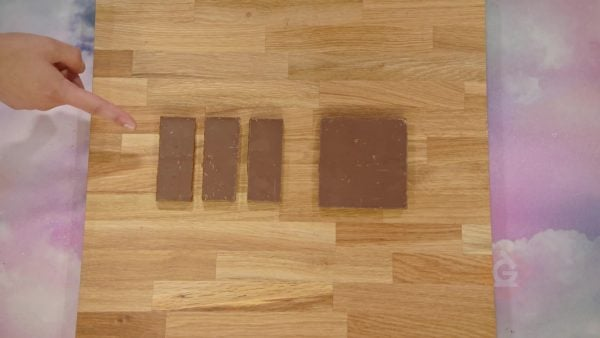Marcos feeds his dog [ggfrac]10/15[/ggfrac] of a treat each day. He can chop the treat into 15 pieces and give the dog 10 of the pieces. This is a lot of work, and Marcos wants to find an easier way. Let’s see if there is an equivalent fraction that would let him cut the dog treat into fewer pieces. If we divide both the numerator and denominator in 1[ggfrac]10/15[/ggfrac] by 5, we get [ggfrac]2/3[/ggfrac]. So, [ggfrac]2/3[/ggfrac] is equivalent to [ggfrac]10/15[/ggfrac]. This saves Marcos a lot of effort because now he only has to cut the dog treat into 3 pieces. Now you try: A recipe calls for [ggfrac]12/16[/ggfrac] of a chocolate bar. What are some equivalent fractions that would make it more efficient to cut up the chocolate?

Chocolate Bars Marcos feeds his dog [ggfrac]10/15[/ggfrac] of a treat each day. He can chop the treat into 15 pieces and give the dog 10 of the pieces. This is a lot of work, and Marcos wants to find an easier way. Let’s see if there is an equivalent fraction that would let him cut the dog treat into fewer pieces. If we divide both the numerator and denominator in 1[ggfrac]10/15[/ggfrac] by 5, we get [ggfrac]2/3[/ggfrac]. So, [ggfrac]2/3[/ggfrac] is equivalent to [ggfrac]10/15[/ggfrac]. This saves Marcos a lot of effort because now he only has to cut the dog treat into 3 pieces. Now you try: A recipe calls for [ggfrac]12/16[/ggfrac] of a chocolate bar. What are some equivalent fractions that would make it more efficient to cut up the chocolate?

## EQUIVALENT FRACTIONS VOCABULARY

Numerator
The number above the line in a fraction, which tells us how many pieces we have.
Denominator
The number below the line in a fraction, which tells us the size of our unit, or how many equal-sized parts are in the whole.
Equivalent
Equal.
Equivalent fractions
Fractions that represent the same amount of the whole.
Double
Multiplied by 2.
Half
Divided by 2.

## EQUIVALENT FRACTIONS DISCUSSION QUESTIONS

### What should we do to turn [ggfrac]1/6[/ggfrac] of a pie into [ggfrac]2/12[/ggfrac]? Does the amount of pie change?

We should cut each slice in half. Now there are twice as many slices and they are twice as small. The amount of pie is the same.

### What are some fractions that are equivalent to [ggfrac]2/3[/ggfrac]?

[ggfrac]4/6[/ggfrac], [ggfrac]6/9[/ggfrac], [ggfrac]8/12[/ggfrac], [ggfrac]10/15[/ggfrac], [ggfrac]12/18[/ggfrac], ...

### How can we check if [ggfrac]5/6[/ggfrac] and [ggfrac]15/18[/ggfrac] are equivalent fractions?

If we multiply 5 by 3, we get 15. If we multiply 6 by 3 we get 18. Both the numerator and denominator are multiplied by 3, so the fractions are equivalent.

### Why do we multiply the numerator and denominator by the same number to find equivalent fractions?

When we cut more slices out of the same amount of a pie, we make more slices (the numerator grows) and at the same time, each slice gets smaller (the denominator grows). Notice that when the numerator and denominator are the same in a fraction, that is the same as 1 whole. Whenever we multiply something by 1, that means we do not change the amount, we just express it in a different way.

### How can we check if [ggfrac]2/5[/ggfrac] and [ggfrac]12/15[/ggfrac] are equivalent fractions?

We can see that 5 × 3 = 15. Then if these are equivalent fractions, we multiply 2 by 3 as well. But 2 × 3 = 6, so [ggfrac]2/5[/ggfrac] and [ggfrac]12/15[/ggfrac] are not equivalent. Or we can see that 2 × 6 = 12. Then we multiply 5 by 6 as well. But 5 × 6 = 30, so [ggfrac]12/15[/ggfrac] is not equivalent to [ggfrac]2/5[/ggfrac]. We could also check using division.
X

## Success

We’ve sent you an email with instructions how to reset your password.
Ok

## Choose Your Free Trial Period### 7 DaysContinue to Lessons### 90 DaysGet 90 days free by inviting other teachers to try it too.

Share with Teachers

## Get 90 Days Free

### By inviting 4 other teachers to try it too.

4 required

Skip, I will use a 7 days free trial

## Thank You!

Enjoy your free 90 days trial